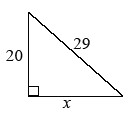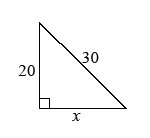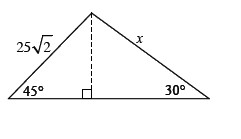### Home > A2C > Chapter 4 > Lesson 4.2.4 > Problem4-126

4-126.

Find the value of $x$.

1.Use the Pythagorean Theorem.

$x = ± 21$

Use the positive side length.

1.See part (a).

$\textit{x}=10\sqrt{5}\approx22.3607$

1.You can use special triangles 30-60-90 and 45-45-90, or solve by using the Law of Sines.

$x = 50$ Remember to check your work.